## Steenrod Algebra

The Steenrod algebra has to do with the Cohomology operations in singular Cohomology with Integer mod 2 Coefficients. For everyandthere are natural transformations of Functorssatisfying:
1.for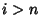.

2.for alland all pairs.

3.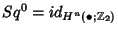.

4. The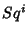maps commute with the coboundary maps in the long exact sequence of a pair. In other words,is a degreetransformation of cohomology theories.

5. (Cartan Relation)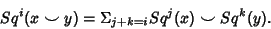6. (Adem Relations) For,7.where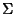is the cohomology suspension isomorphism.

The existence of these cohomology operations endows the cohomology ring with the structure of a Module over the Steenrod algebra, defined to be, where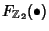is the free module functor that takes any set and sends it to the free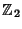module over that set. We think ofas being a gradedmodule, where the-th gradation is given by. This makes the tensor algebrainto a Graded Algebra over.is the Ideal generated by the elementsandfor. This makesinto a gradedalgebra.

By the definition of the Steenrod algebra, for any Space,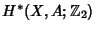is a Module over the Steenrod algebra, with multiplication induced by. With the above definitions, cohomology with Coefficients in the Ring,is a Functor from the category of pairs of Topological Spaces to graded modules over.